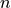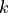# scikit-dimension - Intrinsic dimension estimation in Python¶

scikit-dimension is a Python module for intrinsic dimension estimation.

## What is intrinsic dimension ?¶

Datasets available today frequently have a large number of features and are thus said to be high-dimensional. However many of these features are usually redundant, for example because of linear or non-linear correlations. In such a case it is possible to describe a dataset using a lower number of features. The notion of intrinsic dimension (ID) intuitively refers to the minimal number of features needed to represent a dataset with little information loss.

In the context of the manifold hypothesis, i.e., the hypothesis that data are sampled from an underlying-dimensional manifold, the goal of ID estimation is to recover. Global estimators of ID are based on this hypothesis and return a single estimated dimension for the whole dataset.

When a dataset contains multiple manifolds or regions with different dimensionality (e.g., imagine sampling a line and a sphere and joining them as one dataset), they should be explored using local estimators. The idea behind local ID estimation is to operate in local neighborhoods of each point, where a manifold can be well approximated by its flat tangent space. These local pieces of data are assumed to be close to a uniformly distributed-dimensional ball. In this package, local datasets are simply defined using the-nearest neighbours of each point, and an ID value is thus returned for each datapoint. Such an approach also allows one to repurpose global estimators as local estimators by applying them in each local neighborhood.

## Organization of scikit-dimension¶

scikit-dimension provides a submodule id for intrinsic dimension estimators and datasets to generate benchmark datasets

## Functionalities¶

• estimate global intrinsic dimension
• estimate local intrinsic dimension
• generate toy datasets and widely used synthetic manifolds to benchmark estimators

## Support¶

Feel free to report an issue